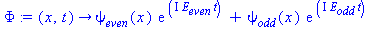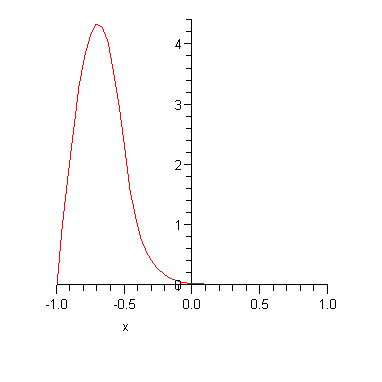Double Well.mw

An example to illustrate how indistinguishable particles can behave as if they are distinguishable

Imagine two electrons bound inside two hydrogen atoms that are far apart. The Pauli exclusion principle says that the two electrons cannot be in the same quantum state because electrons are indistinguishable particles. But the exclusion principle doesn't seem at all relevant when we discuss the electron in a hydrogen atom, i.e. we don't usually worry about any other electrons in the Universe: it is as if the electrons are distinguishable. Our intuition says they behave as if they are distinguishable if they are bound in different atoms but as we shall see this is a slippery road to follow. The complete system of two protons and two electrons is made up of indistinguishable particles so it isn't really clear what it means to talk about two different atoms. For example, imagine bringing the atoms closer together - at some point there aren't two atoms anymore.

You might say that if the atoms are far apart, the two electrons are obviously in very different quantum states. But this is not as obvious as it looks. Imagine putting electron number 1 in atom number 1 and electron number 2 in atom number 2. Well after waiting a while it doesn't anymore make sense to say that "electron number 1 is still in atom number 1". It might be in atom number 2 now because the only way to truly confine particles is to make sure their wavefunction is always zero outside the region you want to confine them in and this is never attainable. We therefore really should treat our two electrons as being indistinguishable from each other, i.e. we are to think of two electrons in the potential of two protons. Let us simplify the situation a little bit by neglecting the interaction between the two electrons - this won't be a bad approximation if the protons are far apart and the electrons are below the ionization energy of 13.6 eV, and in any case it doesn't really affect our argument. The allowed energies of one of the electrons must therefore be approximately equal to the energy levels of an electron in the potential of a single proton (provided the energy is less than the ionization energy). Now the problem is clear - how can both electrons be in (e.g.) the ground state at the same time? Crucially, we are not allowed to appeal to the fact that the electrons are localized on one proton or the other to get round this problem. In the language of quantum mechanics, the energy eigenstates for each electron are not localized on one proton or the other. The initial wavefunction for one electron might be peaked in the region of one proton but after waiting for long enough the wavefunction will evolve to a wavefunction which is not localized at all. In short, the quantum state is completely specified by giving just the electron energies and then it is a puzzle why two electrons can have the same energy (we're also ignoring things like electron spin here but again that is a detail which doesn't affect the main line of the argument). A little thought and you may be able to convince yourself that the only way out of the problem is for there to be two energy levels whose energy difference is too small for us to have ever measured in an experiment. The example presented below is designed to illustrate that this is indeed what is going on.

We'll consider a simplified model. Our system will be two non-interacting particles moving in one dimension. The particles move in the potential illustrated in the figure below: it is an infinite square well with a finite potential barrier in the middle. One can think of putting one particle in the left-hand region and the other in the right-hand region with energies below the barrier height,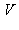. The particles are, for a time, confined however there is always a non-zero tunnelling probability and we cannot therefore say with certainty that the particles remain on one side or the other of the potential barrier. Our goal is to determine the energy eigenvalues and eigenfunctions for the single particle states. If the potential barrier is sufficiently large, then the energy eigenstates will be approximately equal to those of a single particle in an infinite potential well. However, as argued above, we'll encounter the interesting result that there are in fact two slightly non-degenerate energy levels for each energy level of the infinite potential well.Letbe the height of the potential barrier andbe its width. We'll work in units where the Schrödinger equation for the energy eigenstates reads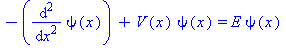We'll also choose our units so that(i.e.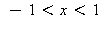). Solving the Schrödinger equation in each of the three regions (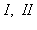and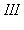) gives: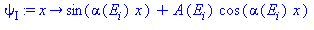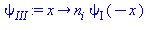where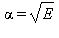,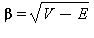. The index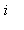labels whether we are considering those eigenstates which are even or odd about the centre of the potential (which I choose to be at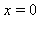). (You should be able to convince yourself that the eigenstates must be either even or odd functions as a result of symmetry.) Consequently,. Note that I have made life a little bit easier by not working with normalized wavefunctions; to get the normalization correct one just has to re-scale by an overall factor but we won't need to bother doing that here because we'll not be computing any probabilities. The boundary conditions are that the wavefunction must vanish for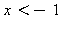and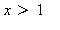and that the wavefunction and its derivative must be continuous at. Implementing these conditions allows us to fixand: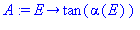and the boundary conditions also lead to the following transcendental equation in the energy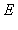.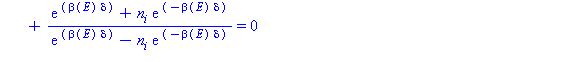In order to do some numerics, let us make a choice for the parameters defining the potential: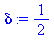Before solving, we'll first consider the energy eigenvalues corresponding to an infinite square well of width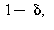which is the result we expect in the case that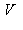tends to infinity. Theth energy eigenstate of the infinte square well is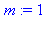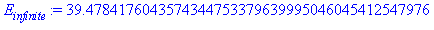There are two corresponding energy eigenstates in our case. The energy of the even eigenstate is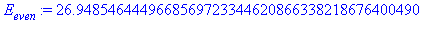and the odd eigenvalue is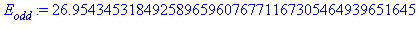Note that the two energy eigenvalues are (a) not very far from the infinite square well result and (b) slightly different from each other. The corresponding energy eigenfunctions are plotted below.The important point is that there are two almost degenerate energy eigenstates for every one energy eigenstate in the corresponding infinite square well. If we had made the potential barrier higher, then the ground state energy would approach that of the infinite square well and the splitting between the energy levels would be even smaller. For example if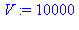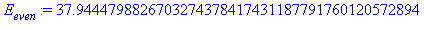Note that I had to go to 50 significant digits to detect the splitting between the two energies (the difference is real, not my numerical error!) You might like to think what determines the size of  the tiny splitting between the energy levels. (Hint: look at the eigenstates in the vicinity of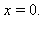)

After working through this exercise you might like to think how things work for an infinite well divided into 3 regions using two finite potential barriers. You might be tempted to think that there are now 4 energy levels for each energy level of the infinite square well corresponding to energy eigenstates that are variously odd/even about the centre of the two potential barriers. However this must be wrong. If it were correct then one could put 4 identical fermions all into the ground state and the Pauli principle would then be violated. You should convince yourself that there are in fact only 3 linearly independent eigenfunctions and hence only 3 energy levels for each energy level of the infinite square well. Now the Pauli principle can still hold: 3 fermions can go into each of the 3 "ground state" levels but the 4th fermion must go into a higher level.

Time evolution

Let's now consider what happens subsequently if we start with the particle on one side of the potential. For the initial wavefunction let us take

which corresponds to a particle located on the left hand side: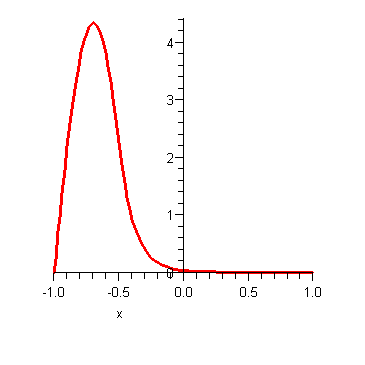This is not an exact energy eigenstate, it is a superposition of two nearly degenerate states and as such it will evolve slowly with time. The below animation shows the subsequent time evolution of the probability density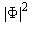which evolves according to